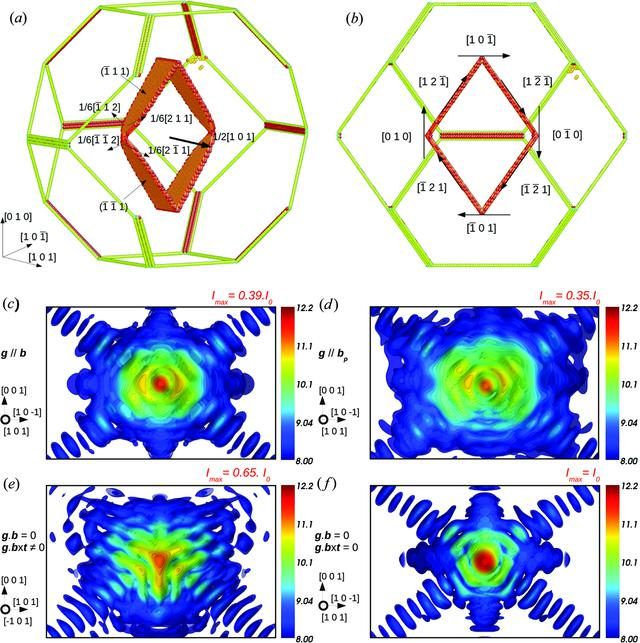disable zoom     view article Figure 8 (a) Relaxed prismatic dislocation loop with b =at the centre of a 30 × 30 × 30 nm Wulff copper crystal. The colour code represents the coordination number, such that only the defective atoms and nanocrystal edges are shown. The loop decomposes into partial dislocations in its (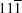) and (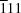) slip planes. (b) The same dislocation loop viewed along the  direction. Calculated CXD pattern for g||b (g = 202) (c), g||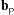(g = 422) (d), g·b = 0 and g·(b × t) ≠ 0 (g =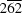) (e), and g·b = 0 and g·(b × t) = 0 (g = 020) (f). The selected area of the reciprocal space is kept to the same value in all figures and is equal to 0.045 × 0.0675 Å−1.JOURNAL OFAPPLIEDCRYSTALLOGRAPHY
ISSN: 1600-5767
Volume 48| Part 3| June 2015| Pages 621-644
Follow J. Appl. Cryst.